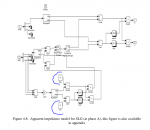#### justkurai

Hello, Guys.

So, I've been looking into modeling and understanding the distance protection.
I checked many articals and stuff.
AND lately I decided to apply and test this distance relay model.
I got the most of the parts, excpet the part of SLG modelI couldn't understand the R(K) and the I(K) over there.

#### simon mugo

Am fumbling between the images trying to understand the circuitry. Could you share a clearer image so that I could try and see how well we can help out?
Thank you!

#### Keonte45

It looks like the R(K) and I(K) refer to the resistance and the imaginary part of the impedance, respectively, at the location of the distance relay, which is labeled as "K" in the diagram. In this model, it appears that the distance relay is being used to protect a transmission line, and the resistance and imaginary impedance at the location of the relay are being used as inputs to the distance relay algorithm.
Distance relays are protection devices that are used to detect faults on transmission lines and other electrical equipment. They work by measuring the electrical quantities (such as voltage, current, and impedance) at the location of the relay and using this information to determine the distance to the fault. The distance relay algorithm uses these measurements to determine whether a fault is present on the transmission line and, if so, to trip the circuit breaker to isolate the fault and protect the electrical equipment.
The R(K) and I(K) values in the diagram represent the resistance and imaginary impedance, respectively, at the location of the distance relay. These values are typically measured or calculated from the voltage and current signals at the relay, and they are used as inputs to the distance relay algorithm to help determine the distance to the fault. In the context of this model, it appears that the R(K) and I(K) values are being used in combination with other electrical quantities (such as the transmission line impedance) to calculate the distance to the fault.